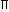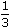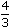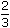Exam Mission

Exam Information| Govt Jobs.| Exam Preparation | Exam Study Material |Exam Date|Material PDF

1. CUBOID
Let length = l, breadth = b and height = h units. Then
1. Volume = (l x b x h) cubic units.
2. Surface area = 2(lb + bh + lh) sq. units.
3. Diagonal = l2 + b2 + h2 units.
2. CUBE
Let each edge of a cube be of length a. Then,
1. Volume = a3 cubic units.
2. Surface area = 6a2 sq. units.
3. Diagonal = 3a units.
3. CYLINDER
Let radius of base = r and Height (or length) = h. Then,
1. Volume = (r2h) cubic units.
2. Curved surface area = (2rh) sq. units.
3. Total surface area = 2r(h + r) sq. units.
4. CONE
Let radius of base = r and Height = h. Then,
1. Slant height, l = h2 + r2 units.
2. Volume =r2hcubic units.
3. Curved surface area = (rl) sq. units.
4. Total surface area = (rl +r2) sq. units.
5. SPHERE
Let the radius of the sphere be r. Then,
1. Volume =r3cubic units.
2. Surface area = (4r2) sq. units.
6. HEMISPHERE
Let the radius of a hemisphere be r. Then,
1. Volume =r3cubic units.
2. Curved surface area = (2r2) sq. units.
3. Total surface area = (3r2) sq. units.
Note: 1 litre = 1000 cm3.